Chapter 5 Class 6 Understanding Elementary Shapes
Concept wise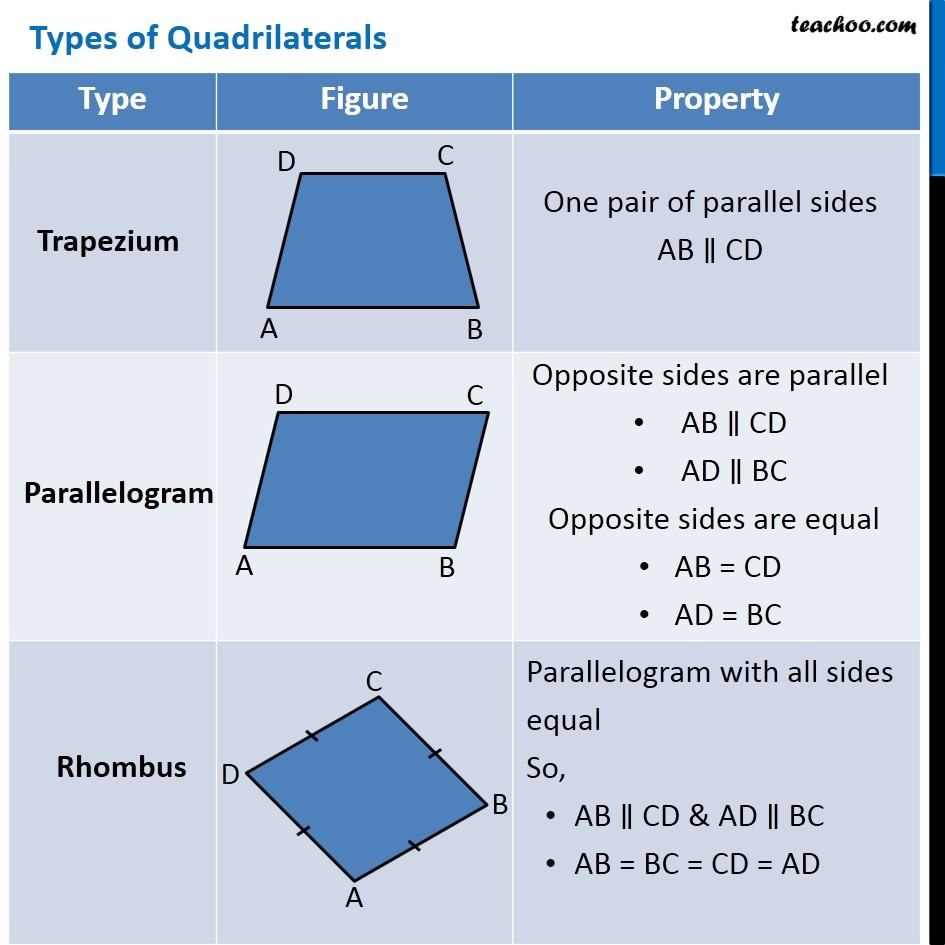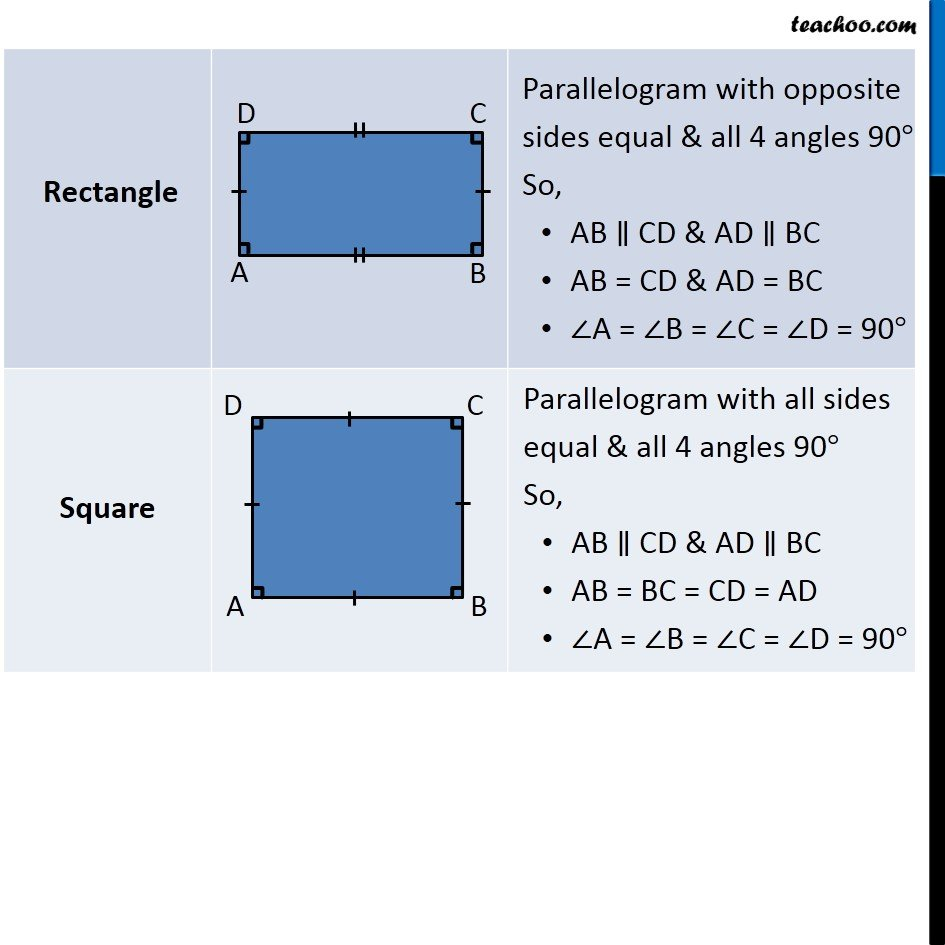## Trapezium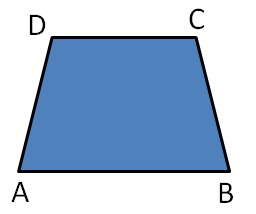One pair of parallel sides

AB ∥ CD

## Parallelogram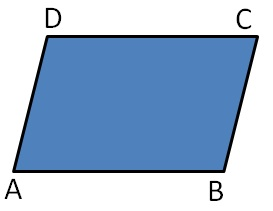Opposite sides are parallel

• AB ∥ CD

Opposite sides are equal

• AB = CD

## Rhombus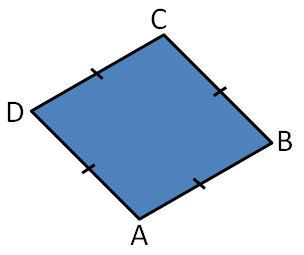Parallelogram with all sides equal

So,

• AB ∥ CD & AD ∥ BC
• AB = BC = CD = AD

## Rectangle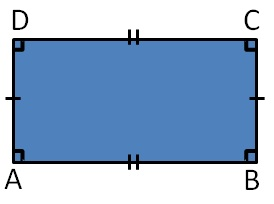Parallelogram with opposite sides equal & all 4 angles 90°

So,

• AB ∥ CD & AD ∥ BC
• AB = CD & AD = BC
• ∠A = ∠B = ∠C = ∠D = 90°

## Square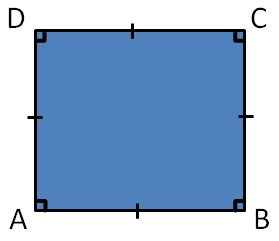Parallelogram with all sides equal & all 4 angles 90°

So,

• AB ∥ CD & AD ∥ BC
• AB = BC = CD = AD
• ∠A = ∠B = ∠C = ∠D = 90°

Introducing your new favourite teacher - Teachoo Black, at only ₹83 per month

### Transcript

One pair of parallel sides AB ∥ CD Two pairs of parallel lines AB ∥ CD AD ∥ BC Opposite two sides are equal AB = CD AD = BC Parallelogram with all sides equal & all 4 angles 90° So, AB ∥ CD & AD ∥ BC AB = BC = CD = AD ∠A = ∠B = ∠C = ∠D = 90° Parallelogram with all sides equal & all 4 angles 90° So, AB ∥ CD & AD ∥ BC AB = CD = AD = BC ∠A = ∠B = ∠C = ∠D = 90° Parallelogram with all sides equal & all 4 angles 90° So, AB ∥ CD & AD ∥ BC AB = BC = CD = AD ∠A = ∠B = ∠C = ∠D = 90°